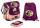# Sides of triangle

Triangle has circumference 42 cm. Side a is 2 times shorter than side b and sice c is 2 cm longer than side a. Determine the sizes of sides of a triangle.

Result

a =  16
b =  8
c =  18

#### Solution:

a+b+c=42
a=2b
c = a+2

a+b+c = 42
a-2b = 0
a-c = -2

a = 16
b = 8
c = 18

Calculated by our linear equations calculator.

Leave us a comment of example and its solution (i.e. if it is still somewhat unclear...):Be the first to comment!#### To solve this example are needed these knowledge from mathematics:

Do you have a linear equation or system of equations and looking for its solution? Or do you have quadratic equation? See also our trigonometric triangle calculator.

## Next similar examples:

1. Center traverseIt is true that the middle traverse bisects the triangle?
2. The largerThe larger of two numbers is nine more than four times the smaller number. The sum of the two numbers is fifty-nine. Find the two numbers.
3. BarbaraBarborka goes to school with backpack that was 2 - times more expensive than a bag slipper. If backpack was 36 euros cheaper it was cost same as bag slipper. How many cost backpack and how many bas slipper?
4. SchoolsThree schools are attended by 678 pupils. To the first attend 21 students more and to the third 108 fewer students than to second school. How many students attend the schools?
5. Boys and girlsThere are 48 children in the sports club, boys are 10 more than girls. How many girls go to the club?
6. Two trainsThere were 159 freight wagons on the railway station creating 2 trains. One had 15 more wagons than the other. How many wagons did each train have?
7. BlackberriesDaniel, Jolana and Stano collected together 34 blackberries. Daniel collected 8 blackberries more than Jolana, Jolana 4 more than Stano. Determine the number blackberries each collected .
8. SummerjobThree students participated in the summerjob. Altogether they earn 1780, -. Peter got a third less than John and Paul got 100,- more than Peter. How much got every one of them?
9. Football seasonDalibor and Adam together scored 97 goals in the season. Adam scored 9 goals more than Dalibor. How many goals scored each?
10. Three figures - numbersThe sum of three numbers, if each is 10% larger than the previous one, is 662. Determine the figures.
11. Football match 4In a football match with the Italy lost 3 goals with Germans. Totally fell 5 goals in the match. Determine the number of goals of Italy and Germany.
12. Linear systemSolve this linear system (two linear equations with two unknowns): x+y =36 19x+22y=720
13. Trees - orchardThe 3/5 trees are apple trees and 1/3 of the trees are cherries. The remaining 5 trees are pear trees. How many trees are in the orchard.
14. Belgium vs ItalyBelgium played a match with Italy and Belgium win by 2 goals. The match fell a total 6 goals. Determine the number of goals scored by Belgium and by Italy.
15. Dining roomThe dining room has 11 tables (six and eight seats). In total there are 78 seats in the dining room. How many are six-and eight-seat tables?
16. Substitutionsolve equations by substitution: x+y= 11 y=5x-25
17. ATC campThe owner of the campsite offers 79 places in 22 cabins. How many of them are triple and quadruple?